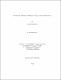## Volatility analysis for high frequency financial data2009
##### Publisher
University of Alabama Libraries
##### Abstract

Measuring and modeling financial volatility are key steps for derivative pricing and risk management. In financial markets, there are two kinds of data: low-frequency financial data and high-frequency financial data. Most research has been done based on low-frequency data. In this dissertation we focus on high-frequency data. In theory, the sum of squares of log returns sampled at high frequency estimates their variance. For log price data following a diffusion process without noise, the realized volatility converges to its quadratic variation. When log price data contain market microstructure noise, the realized volatility explodes as the sampling interval converges to 0. In this dissertation, we generalize the fundamental Ito isometry and analyze the speed with which stochastic processes approach to their quadratic variations. We determine the difference between realized volatility and quadratic variation under mean square constraints for Brownian motion and general case. We improve the estimation for quadratic variation. The estimators found by us converge to quadratic variation at a higher rate.

##### Description
Electronic Thesis or Dissertation
Mathematics